# Regularization in SVMs¶

#### Matt Luther¶

In this post we'll use a toy example to talk about the effect of the regularization parameter C in scikit-learn's support vector machine.

We'll start off by importing a few things we'll need.

In :
%matplotlib inline
import numpy as np
import matplotlib.pyplot as plt
from sklearn.svm import LinearSVC


## Constructing some example samples¶

We'll construct 20 samples by choosing positions randomly from a normal distribution. What we're aiming for is roughly two separated clusters of points, one near the bottom left of the unit square $[0,1] \times [0,1]$, and one near the top right.

We'll label the points in the bottom left as being in class 0, and those in the top right as class 1. Our SVM later will try to separate these classes.

In :
n_samples = 20
features = np.zeros((n_samples,2))
labels = np.zeros((n_samples,1))
mu_1, mu_2 = 0.25, 0.75
std = 0.1
for i in xrange(n_samples/2):
features[i] = np.random.normal(mu_1, std, (1,2))
labels[i] = 0
features[n_samples-1-i] = np.random.normal(mu_2, std,(1,2))
labels[n_samples-1-i] = 1


Since we'll be repeatedly scatter plotting these points, let's build a function for convenience. The function below takes a matrix X whose rows represent the samples, plots the first half as blue circles, and plots the second half as red triangles.

In :
def scatter(X):
plt.scatter(X[:len(X)/2,0], X[:len(X)/2,1], c="lightblue", s = 100, marker='o');
plt.scatter(X[len(X)/2:,0], X[len(X)/2:,1], c="red", s = 100, marker='^');
plt.xlim(0,1), plt.ylim(0,1);


Let's see how our samples look:

In :
scatter(features)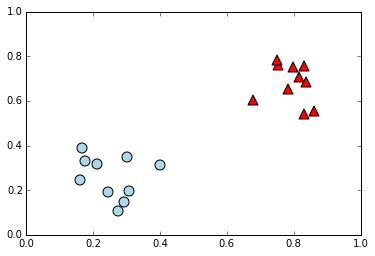They're cleanly separated, which is what we're going for in this toy example. There's some obvious lines we could draw to divide the two clusters.

Our SVM will try to discover such a line. Again, we'll be repeatedly training and plotting the decision boundary for an SVM below, so let's build another function to do this for us. The function below fits scikit-learn's LinearSVC using a feature matrix X and a vector y of corresponding labels. Our function also takes parameters C and alpha. The parameter C corresponds to the regularization parameter C of LinearSVC, and will be the topic of our discussion. The alpha and width parameters are for our convenience in later plotting.

In :
def fit_and_draw_line(X,y,C=1,alpha=1,width=1):
model = LinearSVC(C=C).fit(X,y.reshape(len(X),))
# plot the boundary line
xs = np.arange(0,1,0.01)
cs = model.coef_
ys = -(xs)*(cs/cs) - model.intercept_/cs
plt.plot(xs,ys,'k--',alpha=alpha,linewidth=width);


Let's see the scatter plot and SVM boundary together.

In :
scatter(features)
fit_and_draw_line(features,labels)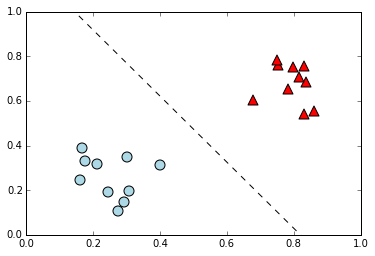We can see that the SVM found a very reasonable boundary line to divide the points into two classes. The samples were very cleanly separated, so this is not surprising. Let's see what happens if we add an outlier.

We'll create a new matrix of features by adding a row for a point near the bottom left cluster, but we'll label this new point with the class associated to the top right cluster.

In :
features_with_outlier = np.r_[features, [[0.25,0.5]]]
labels_with_outlier = np.r_[labels, []]


Let's see what things look like now.

In :
scatter(features_with_outlier)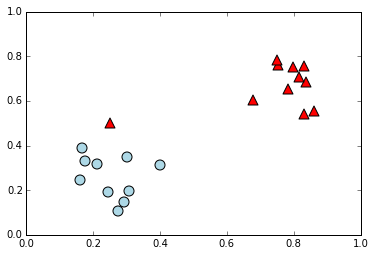Now let's train our SVM again, accounting for this new point. Below, we'll set C = 10000, a very large value compared to the scale of our features. In doing so, we will mimic the behavior of an SVM without any regularization (i.e. one that just tries to find a linear separation at any cost). This will become a bit more clear in the discussion later, but for now, we just observe what kind of boundary we get.

In :
scatter(features_with_outlier)
fit_and_draw_line(features_with_outlier,labels_with_outlier,C=10000)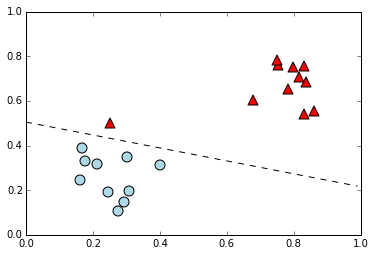Notice that the boundary line still correctly classifies the points, but seems to be taking on some undesirable characteristics. If this new point is really an outlier, then we don't want such a dramatic change in our boundary just to account for it.

Let's see what we get instead if we use C = 1, the default value for the parameter in LinearSVC.

In :
scatter(features_with_outlier)
fit_and_draw_line(features_with_outlier, labels_with_outlier, C=1)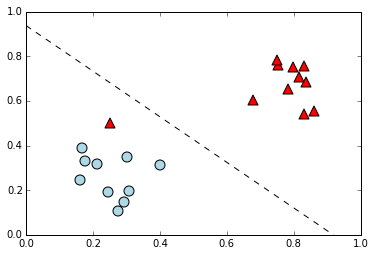This seems like a more reasonable boundary. It has shifted a bit, compared to the boundary line found for the case without the outlier point we added. However, it seems to have made a reasonable trade-off between trying to classify the points correctly, and trying to reasonably "position" the line.

In the next section, we'll talk about the function that LinearSVC tries to minimize, and the role of C in that function.

## Discussion of the optimization function¶

As noted in the scikit-learn docs, LinearSVC fits to our data by trying to find $w,b,\zeta$ minimizing the following function, where $n$ is the number of samples, $$\frac{1}{2}w^{T}w + C \sum_{i=1}^{n} \zeta_i$$ subject to the following constraints for all $i$ $$y_i \left( w^{T} x_i + b \right) \geq 1 - \zeta_i$$ $$\zeta_i \geq 0$$ The vector $w$ is a normal vector to the boundary line, and the term $\frac{1}{2}w^{T}w$ is inversely related to the size of the margin. That is, the distance between the boundary line and the closest point from either class increases when $w^{T} w$ decreases. The terms $\zeta_i$ measure how far the sample $x_i$ is from its "correct" side of the boundary line + margin. That is, $\sum_{i=1}^{n} \zeta_i$ decreases when points are correctly classified by the boundary line, or at least when they are not too far on the wrong side.

Because these two objectives (increasing the margin size and correctly classifying points) conflict, the SVM must make some trade-offs when both are not possible. The C adjusts the weighting of the two terms we discussed above.

Decreasing C means de-emphasizing the $\sum_{i=1}^{n} \zeta_i$ term. Consequently, the $w^T w$ term is emphasized. Consider the extreme case where C is very close to 0. In that case, the function above would be almost entirely determined by $w^T w$, and so would be minimized when $w^T w$ was small, even if the $\zeta_i$ terms were large because they contribute less to the function. This means that when C is smaller, our models worry less about misclassifications. Generally, this can be thought of as increasing the margin size at the expense of a few misclassifications. But notice that in very extreme cases with C nearly 0, the misclassifications are almost entirely ignored and optimizing amounts to selecting anything near the 0 vector. A model trained in that case would be very uninformative.

Increasing C means emphasizing the $\sum_{i=1}^{n} \zeta_i$ term and consequently de-emphasizing the $w^T w$ term. In extreme cases with C very large compared to the scale of our features, this makes the optimization above try to obtain very few misclassifications, possibly at the expense of accepting very large values of $w^T w$ (i.e. a very small margin). That is, such models are very worried about misclassification, to the point that they accept very small margins around the boundary line.

When we chose C = 10000 in our example in the last section, we were asking the model to essentially ignore the $w^T w$ term and just worry about avoiding misclassifications.

When we chose C = 1, we asked the model to allow some misclassifications, and try to pick a line that had bigger margins for the most part.

## How does the boundary vary with C¶

For funzies, we'll show how the boundary line varies as we vary C.

In the first picture, we'll show what happens as C goes from 10000 to 1, that is, between the two examples we showed above. In the picture below, we've plotted several boundary lines. The fainter lines correspond to the higher values of C, and the darker lines correspond to the values closer to 1.

In :
scatter(features_with_outlier)
alpha = 0.1
for c in [10000, 3000, 1000, 300, 100, 30, 10, 3, 1]:
fit_and_draw_line(features_with_outlier, labels_with_outlier, C=c, alpha=alpha, width=alpha*3)
alpha += 0.1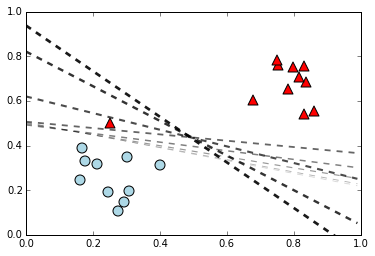We can see in this last picture that the lines rotate to a more vertical position as C moves from 10000 toward 1. The lines seem to worry less and less about misclassifying the outlier point, and shift toward the line we found for our original samples before we included the outlier.

We might think that this trend would continue if we continued to shrink C toward 0. However, remember that as C approaches 0, the optimization we discussed will eventually disregard the $\sum_{i=1}^{n} \zeta_i$ term almost entirely. Consequently, as C approaches 0, the SVM loses interest in classifying the points, and worries almost entirely about minimizing $w^T w$.

Let's see what this looks like. Below, we show what happens as C moves from 1 toward 0. The fainter lines correspond to lines with C near 1, and the darker lines correspond to lines with C near 0.

We'll expand the graphing window a bit to see what happens more easily.

In :
scatter(features_with_outlier)
alpha = 0.2
for c in [1, 0.3, 0.1, 0.03, 0.01]:
fit_and_draw_line(features_with_outlier, labels_with_outlier, C=c, alpha=alpha, width=alpha*3)
alpha += 0.2
plt.xlim(-0.1,1.1)
plt.ylim(-0.5,1.1)

Out:
(-0.5, 1.1)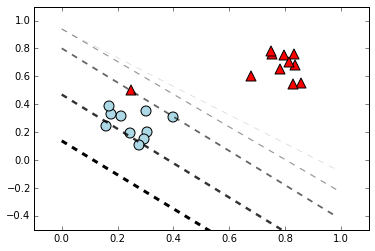We can see that the lines for C closer to 0 seem to disregard the features, placing all of the samples on the same side.

## Choosing the right C¶

In our toy example above, values of C near the default value of 1 seemed to be most appropriate. In general, this might not be the case. The value of C and the trade-offs involved need to be considered indepedently for different problems. The "correct" value of C for a particular learning problem can be chosen by trying various values for C and choosing the one most appropriate for the task at hand. In our post on classification in the iris data set with an SVM, we chose C in this way, using a validation step where we scored the models by accuracy as C varied.